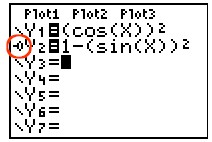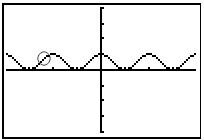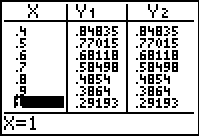Verifying Trig Identities

If you need to determine if an equation is a trigonometric identity, you can use your graphing calculator.  A "formal proof" of a trigonometric identity is done algebraically.  The graphing calculator, however, can be used to "check" your work.

 Determine if   cos2 x = 1 - sin2 x   is a trig identity:

 Enter the left side in Y1. Enter the right side in Y2. Set the bubble animation for the second graph.Choose ZOOM #7 ZTRigIf the bubble runs over the same graph as the first plotting, the equation is a trig identity.2nd GRAPH (TABLE)The TABLE can also be used to check to see if the results are the same from both graphs.  Arrow up and down to see if the values listed under columns Y1 and Y2 remain the same. Adjust your table through 2nd WINDOW (TBLSET) to see more varied values.

Answer:  Yes,  cos2 x = 1 - sin2 x   is a trig identity.